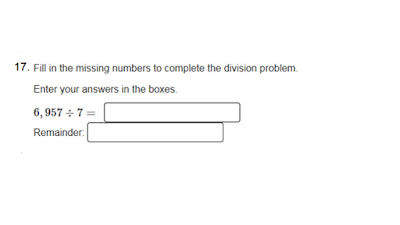Tuesday, December 6, 2016

PARCC Released Math Items: Why Review Only One Problem At a Time?

Today, after the 4th Grader I'm tutoring finished our work together, about calculating a long division problem with remainders,  we looked at this one 2015 Released PARCC math test item:Together we talked about how some questions on the PARCC don't have you select an answer from a list of possibilities. You have to do the calculations yourself and enter the numbers into a box.  We also noticed that the remainder would be listed in a separate box.
Then we discussed:
"If you want to check your division quotient to make sure it is correct, what would you do?"
"I'd multiply."
"Tell me what to do, and I'll check our quotient."
"Multiply 993 times 7."
"Okay, so that is only 6,951, so I guess we made a mistake."
"Nope, you have to add the remainder of 6 to that answer and then you'll have 6,957. So we know that I am correct."

My point is that by only looking at one problem, we reviewed how to do a long division problem with a remainder, reviewed how to check your answer, and realized that sometimes I won't be choosing from a list of answers- that I will need to enter my answer into 2 boxes (computer format).
Smiles,
Deborah